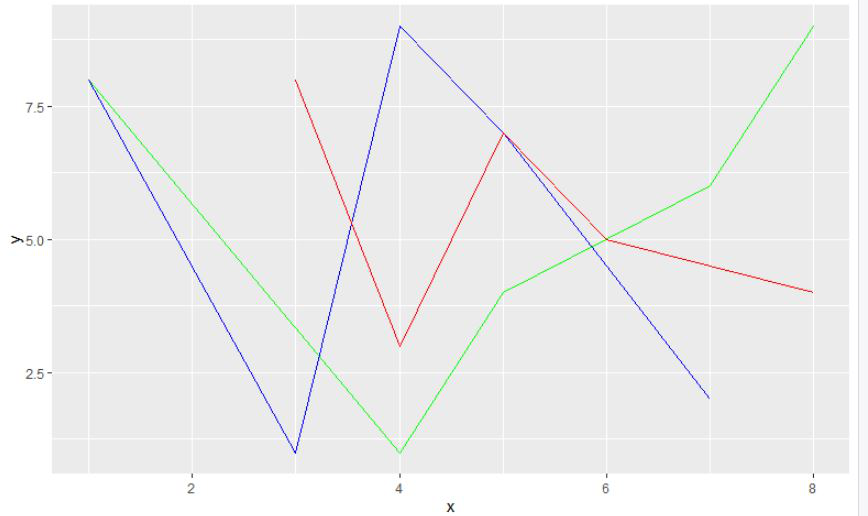GFG App
Open AppBrowser
Continue

# How to Combine Multiple ggplot2 Plots in R?

In this article, we will discuss how to combine multiple ggplot2 plots in the R programming language.

## Combining multiple ggplot2 plots using ‘+’ sign to the final plot

In this method to combine multiple plots, here the user can add different types of plots or plots with different data to a single plot, the user only needs to use the ‘+’ sing to combine the plot with each plot to the final plot, and further, the final plot will be a combination of multiple plots in the single ggplot2 plot in the R programming language.

Syntax:

ggplot(NULL, aes(x, y)) +geom_line(data 1)+geom_line(data 2)+……+geom_line(data n)

Example 1:

In this example, we will be combining 3 ggplot2 line plots with different data using the ‘+’ sign form combining them into a single plot in the R programming language.

## R

 `# load the package ` `library``(``"ggplot2"``) ` ` `  `# create dataframe ` `gfg1 < -``data.frame``(x=``c``(4, 5, 1, 7, 8),  ` `                   ``y=``c``(1, 4, 8, 6, 9)) ` ` `  `# create dataframe ` `gfg2 < -``data.frame``(x=``c``(5, 1, 4, 3, 7), ` `                   ``y=``c``(7, 8, 9, 1, 2)) ` ` `  `# create dataframe ` `gfg3 < -``data.frame``(x=``c``(4, 8, 6, 3, 5), ` `                   ``y=``c``(3, 4, 5, 8, 7)) ` ` `  `# plot the data with dataframes with green ` `# red and blue colors ` `gfg_plot < -``ggplot``(``NULL``, ``aes``(x, y)) +  ` `geom_line``(data=gfg1, col=``"green"``) + ` `geom_line``(data=gfg2, col=``"blue"``)+ ` `geom_line``(data=gfg3, col=``"red"``) ` ` `  `# display the plot ` `gfg_plot `

Output:Example 2:

In this example, we will be combining 3 ggplot2 line plots and 2 scatter ggplot2 plots a total of 5 plots with different data using the ‘+’ sign form combining them into a single plot in the R programming language.

## R

 `# load the package ` `library``(``"ggplot2"``) ` ` `  `# create 5 dataframes ` `gfg1 < -``data.frame``(x=``c``(4, 5, 1, 7, 8),  ` `                   ``y=``c``(1, 4, 8, 6, 9)) ` `gfg2 < -``data.frame``(x=``c``(5, 1, 4, 3, 7), ` `                   ``y=``c``(7, 8, 9, 1, 2)) ` `gfg3 < -``data.frame``(x=``c``(4, 8, 6, 3, 5),  ` `                   ``y=``c``(3, 4, 5, 8, 7)) ` `gfg4 < -``data.frame``(x=``c``(8, 6, 7, 2, 1),  ` `                   ``y=``c``(8, 6, 4, 1, 9)) ` `gfg5 < -``data.frame``(x=``c``(6, 1, 6, 5, 4), ` `                   ``y=``c``(6, 8, 7, 6, 4)) ` ` `  `# plot the data with 5 dataframes ` `# with 5 different colors ` `gfg_plot < -``ggplot``(``NULL``, ``aes``(x, y)) +  ` `geom_line``(data=gfg1, col=``"green"``) + ` `geom_line``(data=gfg2, col=``"blue"``)+ ` `geom_line``(data=gfg3, col=``"red"``)+ ` `geom_point``(data=gfg4, col=``"purple"``) +  ` `geom_point``(data=gfg5, col=``"black"``) ` ` `  `# display the plot ` `gfg_plot `

Output:My Personal Notes arrow_drop_up